# Topic 5 Applications of Integrals

## 5.1 Area between Curves

To find the area between curves, we identify a variable and use the differential in that variable as the width and the distance between the curve as the height and approximate the area using the Riemann sum of such a partition.

If the curves are given by $$y=f(x)$$ and $$y=g(x)$$ with $$a\le x\le b$$, then the area between the curves may be set up as $\text{Area Between Curves}=\int_a^b|f(x)-g(x)|\mathrm{d}x$ Because the distance in $$y$$ direction is easier to calculate.

If the curves are given by $$x=p(y)$$ and $$x=q(y)$$ with $$c\le y\le d$$, then the area between the curves may be set up as $\text{Area Between Curves}=\int_c^d|p(y)-q(y)|\mathrm{d}y$ Because the distance in $$x$$ direction is easier to calculate.

The absolute value sign can be removed by breaking the area into sub-areas.

Exercise 5.1 Sketch the graph of curves $$y=4-x^2$$, $$y=2x+1$$, $$x=-2$$, and $$x=0$$, and find the area enclosed by those curves.

Solution.

The graph of the curves shows that a vertical rectangular slice of the region has an area $$[(4-x^2)-(2x+1)]\mathrm{d} x$$.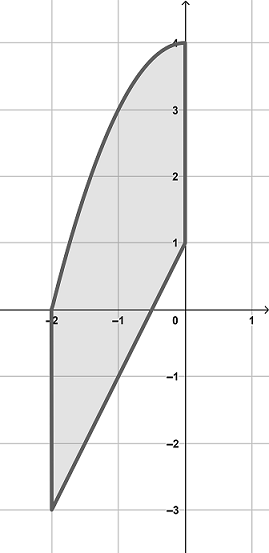Area Between Two Curves

Then the area of the region can be calculated by the definite integral $\int_{-2}^0[(4-x^2)-(2x+1)]\mathrm{d} x=(-\frac{x^3}{3}-x^2+3x)|_{-2}^0=\frac{22}{3}.$

Exercise 5.2 Sketch the graph of curves $$y=x^2-2$$ and $$y=2$$, and find the area enclosed by those curves.

Solution.

Solve the system of equations $\begin{cases} y=x^2-2\\ y=2, \end{cases}$ we get two intersection points $$(-2, 2)$$ and $$(2, 2)$$. Over the interval $$[-2, 2]$$, $$x^2-2\le 2$$. Then the area of the region can be calculated by the definite integral $\int_{-2}^2[2-(x^2-2)]\mathrm{d} x=2\int_{0}^2(4-x^2)\mathrm{d} x =2(4x-\frac{x^3}{3})|_{0}^2=\frac{32}{3}.$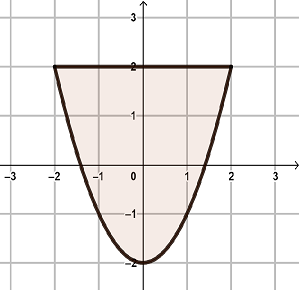Area Between Two Curves

Exercise 5.3 Sketch the graph of curves $$y=x^2+x-2$$ and $$y=1-x$$, and find the area enclosed by those curves.

Solution.

Solve the system of equations $\begin{cases} y=x^2+x-2\\ y=1-x, \end{cases}$ we get two intersection points $$(-3, 4)$$ and $$(1, 0)$$. Since the function $$(x^2+x-2)\le (1-x)$$ for $$-3\le x\le 1$$, the area of the region can be calculated by the definite integral $\int_{-3}^1[(1-x)-(x^2+x-2)]\mathrm{d} x=(3x-\frac{x^2}{2}-\frac{x^3}{3})|_{-3}^1=\frac{32}{3}.$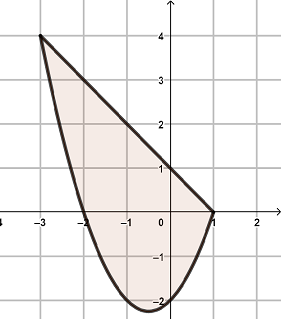Area Between Two Curves

Exercise 5.4 Sketch the graph of curves $$y=\cos x$$ and $$y=2\sin x\cos x$$ with $$-\pi/2\le x\le \pi/2$$ and find the area between those curves.

Solution.

Solve the system of equations $\begin{cases} y=\cos x\\ y=2\sin x\cos x, \end{cases}$ we get three intersection points $$(-\pi/2, 0)$$, $$(\pi/6, \sqrt{3}/2)$$ and $$(\pi/2, 0)$$. The graph of the functions are shown below.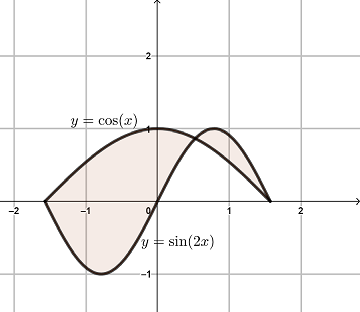Area Between Two Curves

The area of the region can be calculated by the sum of two definite integral \begin{aligned} &\int_{-\pi/2}^{\pi/2}|\cos x-2\sin x\cos x|\mathrm{d} x\\ =&\int_{-\pi/2}^{\pi/6}[\cos x-2\sin x\cos x]\mathrm{d} x+\int_{\pi/6}^{\pi/2}[2\sin x\cos x-\cos x]\mathrm{d} x\\ =&-\frac14(1-2\sin x)^2\big|_{-\pi/2}^{\pi/6}+\frac14(2\sin x-1)\big|_{\pi/6}^{\pi/2}\\ =&\frac94+\frac14\\ =&\frac52. \end{aligned}

Exercise 5.5 Sketch the graph of curves $$y=|x|$$ and $$y=-x^2+2$$ and find the area enclosed by those curves.

Solution.

Solve the system of equations $\begin{cases} y=|x|\\ y=-x^2+2, \end{cases}$ we get three intersection points $$(-1, 1)$$, and $$(1, 1)$$. The graph of the functions are shown below.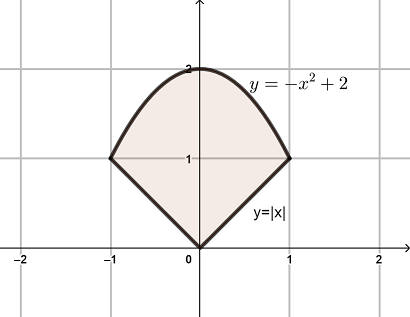Area Between Two Curves

The area of the region can be calculated by the definite integral \begin{aligned} &\int_{-1}^{1}||x|-(-x^2+2)|\mathrm{d} x\\ =&2\int_{0}^{1}||x|-(-x^2+2)|\mathrm{d} x\\ =&2\int_{0}^{1}(-x^2+2-x)\mathrm{d} x\\ =&2(-\frac{x^3}{3}-\frac{x^2}{2}+2x)\big|_0^1\\ =&\frac73. \end{aligned}

Exercise 5.6 Sketch the graph of curves $$x+y^2=5$$ and $$y^2=x+3$$ and find the area enclosed by those curves.

Solution.

Solve the system of equations $\begin{cases} x+y^2=5\\ y^2=x+3, \end{cases}$ we get three intersection points $$(1, -2)$$, and $$(1, 2)$$. The graph of the functions are shown below.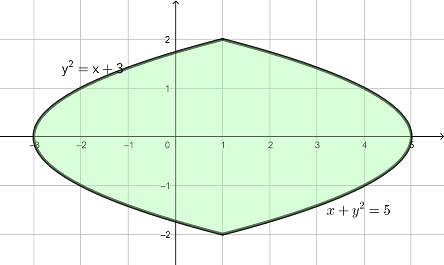Area Between Two Curves

The area of the region can be calculated by the definite integral \begin{aligned} &\int_{-2}^{2}|(5-y^2)-(y^2-3)|\mathrm{d} y\\ =&2\int_{0}^{2}(-2y^2+8)\mathrm{d} y\\ =&2(-\frac{2y^3}{3}+8y)\big|_0^2\\ =&\frac{64}{3}. \end{aligned}

Exercise 5.7 Sketch the graph of curves $$y=x$$ and $$x=y^2-y-3$$ and find the area enclosed by those curves.

Solution.

Solve the system of equations $\begin{cases} y=x\\ x=y^2-y-3, \end{cases}$ we get three intersection points $$(-1, -1)$$, and $$(3, 3)$$. The graph of the functions are shown below.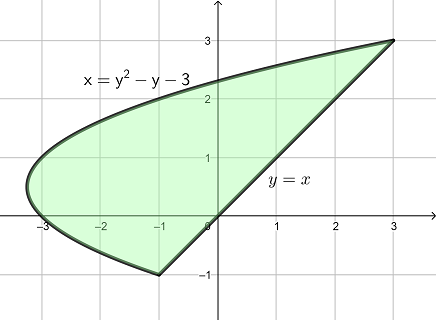Area Between Two Curves

The area of the region can be calculated by the definite integral \begin{aligned} &\int_{-1}^{3}|y-(y^2-y-3)|\mathrm{d} y\\ =&\int_{-1}^{3}(-y^2+2y+3)\mathrm{d} y\\ =&(-\frac{y^3}{3}+y^2+3y)\big|_{-1}^3\\ =&\frac{32}{3}. \end{aligned}

Exercise 5.8 Sketch the graph of curves $$x=y^4$$ and $$y^2=2-x$$ and find the area enclosed by those curves.

Solution.

Solve the system of equations $\begin{cases} x=y^4\\ y^2=2-x, \end{cases}$ we get three intersection points $$(1, -1)$$, and $$(1, 1)$$. The graph of the functions are shown below.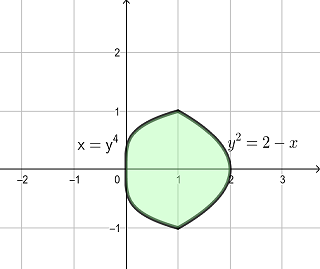Area Between Two Curves

The area of the region can be calculated by the definite integral \begin{aligned} &\int_{-1}^{1}|y^4-(2-y^2)|\mathrm{d} y\\ =&2\int_{0}^{1}(2-y^2-y^4)\mathrm{d} y\\ =&2(-\frac{y^5}{5}-\frac{y^3}{3}+2y)\big|_{0}^1\\ =&\frac{44}{15}. \end{aligned}

Exercise 5.9 Sketch the graph of curves $$y=x^2-1$$ and $$y=x^3+x^2-x-1$$ and find the area enclosed by those curves.

Solution.

Let $$D(x)=(x^2-1)-(x^3+x^2-x-1)=-x^3+x$$. Solve $$D(x)=0$$, we get three solutions $$x=-1$$, $$x=0$$, and $$x=1$$. Using test point method or algebraic method, we find that $$D(x)<0$$ for $$-1<x<0$$ and $$D(x)>0$$ for $$0<x<1$$. The graph of the functions are shown below.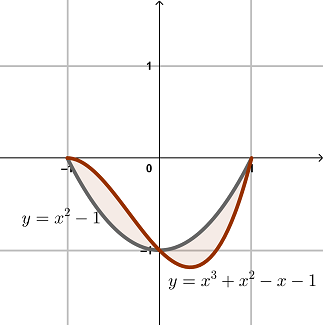Area Between Two Curves

The area of the region can be calculated by the sum of two definite integral \begin{aligned} &\int_{-1}^{1}|-x^3+x|\mathrm{d}x\\ =&2\int_{0}^{1}(-x^3+x)\mathrm{d} x =&2(-\frac{x^4}{4}+\frac{x^2}{2})\big|_{0}^1\\ =&\frac12. \end{aligned}

Exercise 5.10 Sketch the graph of curves $$x-2y=0$$ and $$y^3-y^2=x$$ and find the area enclosed by those curves.

Solution.

Since $$x$$ is easier to solve, it is better to use the sum of areas horizontal rectangles, that is the area is given by $\int_a^b|x_R(y)-x_L(y)|\mathrm{d}y.$ Solve for $$x$$ from the equations of the curves and set $$D(y)=(y^3-y^2)-2y=y^3-y^2-2y$$. Solve $$D(y)=0$$, we get three solutions $$y=-1$$, $$y=0$$, and $$y=2$$. Using test point method or algebraic method, we find that $$D(y)>0$$ for $$-1<y<0$$ and $$D(y)<0$$ for $$0<y<2$$. The graph of the functions are shown below.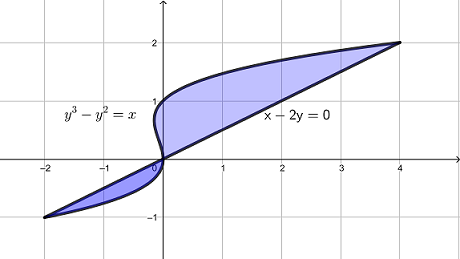Area Between Two Curves

The area of the region can be calculated by the sum of two definite integral \begin{aligned} &\int_{-1}^{2}|y^3-y^2-2y|\mathrm{d} y\\ =&\int_{-1}^{0}(y^3-y^2-2y)\mathrm{d} y-\int_{0}^{2}(y^3-y^2-2y)\mathrm{d} y\\ =&(\frac{y^4}{4}-\frac{x^3}{3}-y^2)\big|_{-1}^0-(\frac{y^4}{4}-\frac{x^3}{3}-y^2)\big|_{0}^2\\ =&\frac{5}{12}+\frac83\\ =&\frac{37}{12}. \end{aligned}